# Hex, Bugs and More Physics | Emre S. Tasci

a blog about physics, computation, computational physics and materials…

## Some “trivial” derivations

### December 4, 2007 Posted by Emre S. Tasci

Information Theory, Inference, and Learning Algorithms by David MacKay, Exercise 22.5:

A random variable x is assumed to have a probability distribution that is a mixture of two Gaussians,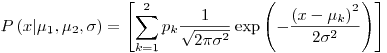where the two Gaussians are given the labels k = 1 and k = 2; the prior probability of the class label k is {p1 = 1/2, p2 = 1/2};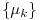are the means of the two Gaussians; and both have standard deviation sigma. For brevity, we denote these parameters byA data set consists of N points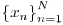which are assumed to be independent samples from the distribution. Let kn denote the unknown class label of the nth point.

Assuming thatand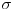are known, show that the posterior probability of the class label kn of the nth point can be written asand give expressions for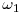and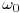.

Derivation: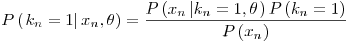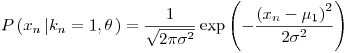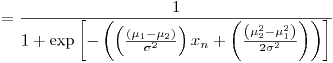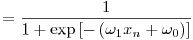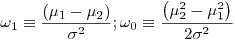Assume now that the meansare not known, and that we wish to infer them from the data. (The standard deviationis known.) In the remainder of this question we will derive an iterative algorithm for finding values forthat maximize the likelihood,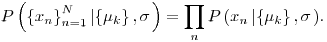Let L denote the natural log of the likelihood. Show that the derivative of the log likelihood with respect to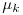is given by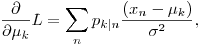where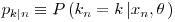appeared above.

Derivation: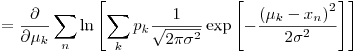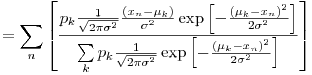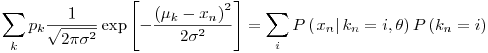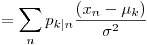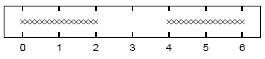Assuming that=1, sketch a contour plot of the likelihood function as a function of mu1 and mu2 for the data set shown above. The data set consists of 32 points. Describe the peaks in your sketch and indicate their widths.

Solution:

We will be trying to plot the functionif we designate the function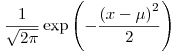as p[x,mu] (remember that=1 and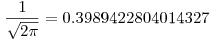),then we have:And in Mathematica, these mean:

mx=Join[N[Range[0,2,2/15]],N[Range[4,6,2/15]]]
Length[mx]
ListPlot[Table[{mx[[i]],1},{i,1,32}]]p[x_,mu_]:=0.3989422804014327` * Exp[-(mu-x)^2/2];
pp[x_,mu1_,mu2_]:=.5 (p[x,mu1]+p[x,mu2]);
ppp[xx_,mu1_,mu2_]:=Module[
{ptot=1},
For[i=1,i<=Length[xx],i++,
ppar = pp[xx[[i]],mu1,mu2];
ptot *= ppar;
(*Print[xx[[i]],"\t",ppar];*)
];
Return[ptot];
];

Plot3D[ppp[mx,mu1,mu2],{mu1,0,6},{mu2,0,6},PlotRange->{0,10^-25}];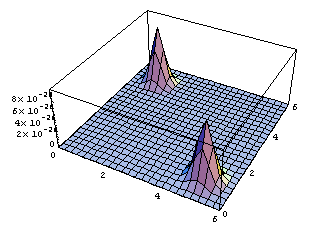ContourPlot[ppp[mx,mu1,mu2],{mu1,0,6},{mu2,0,6},{PlotRange->{0,10^-25},ContourLines->False,PlotPoints->250}];(*It may take a while with PlotPoints->250, so just begin with PlotPoints->25 *)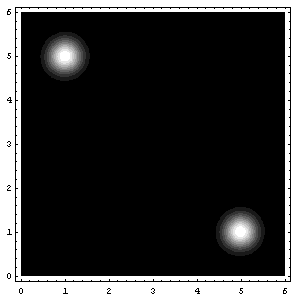That’s all folks! (for today I guess 8) (and also, I know that I said next entry would be about the soft K-means two entries ago, but believe me, we’re coming to that, eventually 😉

Attachments: Mathematica notebook for this entry, MSWord Document (actually this one is intended for me, because in the future I may need them again)